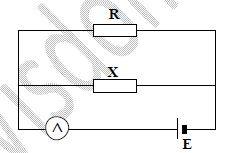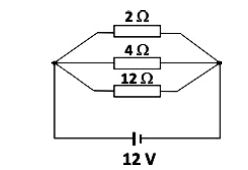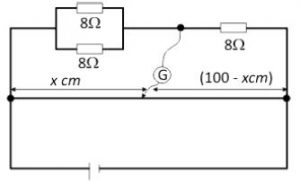Physic Tutorials

How to solve questions on current of electricity for UTME

How to solve questions on current of electricity for UTME

Question 1

A working electric motor takes a current of 1.5A when the p.d. across it is 250V. If its efficiency is 80%, the power output is{UTME 2001}
A. 469.0W        B. 300.0W        C. 4.8W          D. 133.0W

Solution

Efficiency = (power output / power input)%

power input = IV = 1.5*250 = 375W

80/100 = power output / 375

power output = 80*375 / 100 = 300 W

B is the correct option

Question 2

A bread toaster uses a current of 4A when plugged in a 240 volts line. It takes one minute to toast slices of bread. What is the energy consumed by the toaster?{UTME 2001}
A. 3.60 x 103J       B. 5.76 x 104J      C. 1.60 x 102J        D. 1.60 x 104J

Solution

one minute is 60 seconds

Energy consumed = IVt = 4*240*60 = 57600 J = 5.76 x 104 J

B is the correct answer

Question 3In the circuit diagram above, the ammeter reads a current of 3A when R is 5Ω and 6A when R is 2Ω. Determine the value of x.{UTME 2001}
A. 10Ω            B. 8Ω         C. 4Ω            D. 2Ω

Solution

X and R are in parallel

Effective resistance = (X*R)/(R+X)

When current is 3A, the effective resistance = 5X /(5+X)

V = IR

V = 3[5X /(5+X)]

when current is 6A, the effective resistance = 2X /(2+X)

V = IR

V = 6[2X /(2+X)]

Since R and X are in parallel, the same voltage will be applied across the two resistors

3[5X /(5+X)] = 6[2X /(2+X)]

15X /(5+X) = 12X/(2+X)

15/(5+X) = 12/(2+X)

cross multiply

15(2+x) = 12(5+x)

30 +15x = 60 + 12x

3x = 30

x = 10Ω

A is the correct answer

Question 4

A cell of emf 1.5V is connected in series with a 1Ω resistor and a current of 0.3A flows through the resistor. Find the
internal resistance of the cell.{UTME 2013)
A. 1.0 Ω        B. 4.0 Ω          C. 3.0 Ω            D. 1.5 Ω

Solution

E = I(R+r)

1.5 = 0.3(1 + r)

1 + r = 1.5/0.3

1 + r =5

r = 5 – 1 = 4Ω

B is the correct option

Question 5

Which of the following obeys ohms law?{UTME 2013}
A. Glass             B. Glectrolytes            C. Metals                D. Diode

Solution

Ohm’s law is about metallic material only provided the temperature and all other physical factors rmain constant.

C is the correct option

Question 6

Six identical cells, each of e.m.f. 2V are connected as shown above. The effective e.m.f. of the cell is{UTME 2012}
A. 0V        B. 4V        C. 6V          D. 12V

Solution

cells arranged in series will have effective emf of

E = E1 + E2 + E3 +…

E = 2+2+2+2+2+2 = 12 V

D is the correct option

Question 7In the circuit above, three resistors, 2Ω, 4 Ω and 12 Ω are connected in parallel and a 12 V battery is connected across the combination. The current flowing through the 12 Ω resistor is{UTME 2011}
A. 9.6 A             B 14.4 A               C. 1.0 A               D. 3.2 A.

Solution

The current flowing through the 12 Ω resistor will be, V =IR

Since they are all in parallel, they will have the same voltage across them,

12 = I*12

I = 12/12 = 1A

C is the correct option

Question 8The diagram above shows a balanced metre bridge, the value of x is{UTME 2010}
A. 66.7 cm              B. 25.0 cm              C. 33.3 cm                 D. 75.0 cm.

Solution

R1 /R2 = L1 / L2

R1, the resistors are in parallel, so the effective resistance = 8*8/(8+8) = 64/16 =4Ω

4 / x = 8 / (100-x)

4(100-x) = 8x

400 -4x = 8x

12x = 400

x = 400 / 12 = 33.33 cm

C is the correct option

Question 9In the diagram above, a 200 W bulb is lighted by a 240 V a.c mains supply. If 1kWh is sold at N 40, the cost of keeping the bulb lighted for a day is{UTME 2010}

A. N 192.00              B. N 1.92                    C. N 19.20                    D. N 1,920.00.

Solution

Energy consumed = power(KW) * time(hour)

Energy consumed = 200/1000 * (a day = 24hrs) = 0.2*24 = 4.8KWh

1kWh is sold at N 40

4.8KWh will be N40*4.8 = N192.00

A is the correct option

Bolarinwa Olajire

A lecturer, Educationist, PhD student at FUNAAB, and a Blogger.

Related Articles

1.Pinky says:

thank you.. it helped

2.anisiji wisdom says:

a house hold refigirator consumes eletrical energy at the rate of 200w if electricity cost 5 kobo / 3.6×10^6J energy calculate the cost of operating of appliance for 30 days pls help me solve this equation i need it now

1.Bolarinwa Olajire says:

Energy = power x time (secs) = 200 x 30 x 24 x 60 x 60 = 518400000J